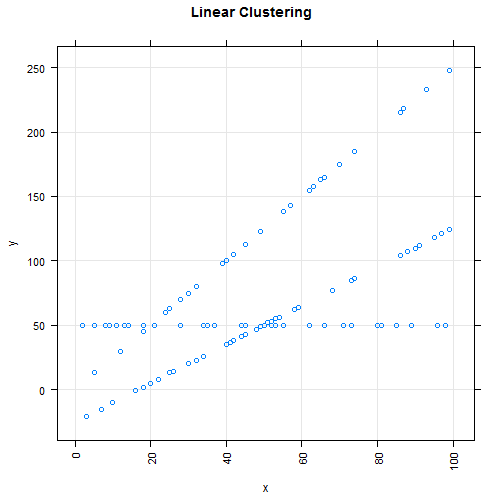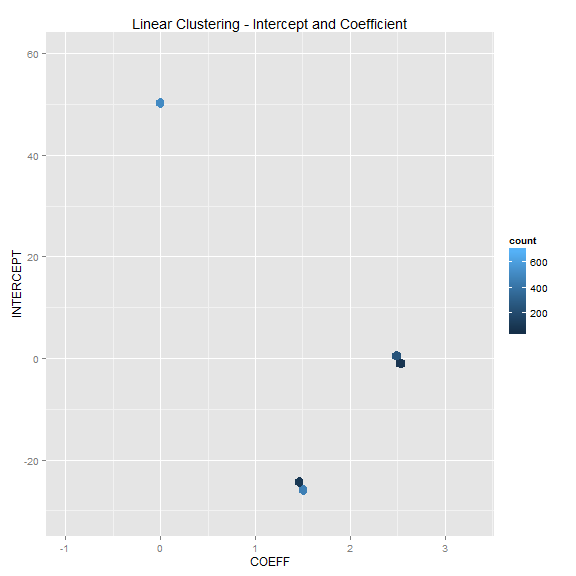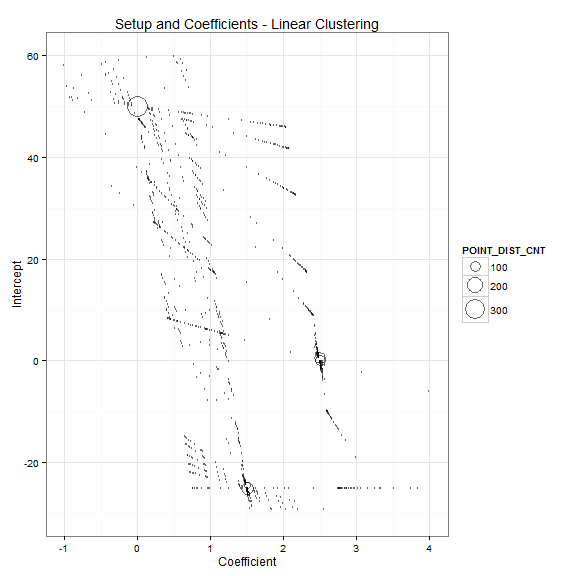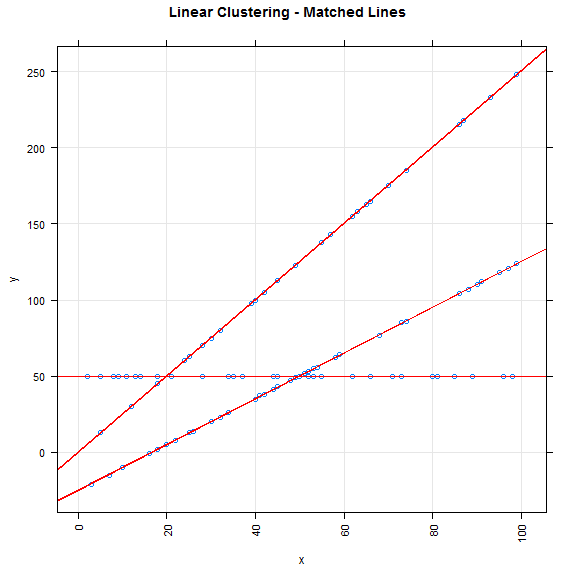# Linear Clustering

Performed on So Jun 19 16:11:24 2016.

## Get Data

``````df <-  dbGetQuery(jdbcConnection,
"with rn as (
select DBMS_RANDOM.VALUE(0,1) rn1,
DBMS_RANDOM.VALUE(0,1) rn2
from dual connect by level <= 100
), rate as (
select round(rn1*100) vol,
floor(rn2*3) tarif
from rn)
select
tarif line_id,
vol x,
round(
case when tarif = 0 then 50
when tarif = 1 then vol* 2.5
when tarif = 2 then 50 + (vol-50) * 1.5 end) as y
from rate
")

``````
``````##    X   Y
## 1 30  75
## 2  5  50
## 3 40  35
## 4 66 165
## 5 57 143
## 6 34  50
``````

## Graph Data## Save File

The data is stored in the file linear_clustering_avd.csv

LINE_ID use case 1,2

X,Y coordinates of the point

Example

``````##  TRUE
``````

## Calculate the Intercept and Coefficient of the Lines

Following formulas are used: i - intercept; c - coefficient

A(y) = i + c * A(x)

B(y) = i + c * B(x)

A(y) - B(y) = c * (A(x) - B(x))

c = (A(y) - B(y)) / (A(x) - B(x))

i = A(y) - c * A(x)

``````df.coeff <-  dbGetQuery(jdbcConnection,
"-- derive intercept + coeff
with derive as (
select a.x a_x, b.x b_x, a.x - b.x delta_x,
a.y a_y, b.y b_y, a.y - b.y delta_y
where a.x > b.x + 5  /* minimum line length */
), derive2 as (
select
a_x,  b_x,a_y, b_y,
round(DELTA_Y / DELTA_X,6) coeff
from derive
), clust  as (
select   a_x,  b_x,a_y, b_y,
a_y - coeff * a_x as intercept,
coeff
from derive2)
select A_X, B_X, A_Y, B_Y, INTERCEPT, COEFF from clust")

``````
``````##   A_X B_X A_Y B_Y   INTERCEPT      COEFF
## 1  99  93 124 233 1922.500033 -18.166667
## 2  99  91 124 112  -24.500000   1.500000
## 3  99  90 124 110  -30.000044   1.555556
## 4  99  90 124 110  -30.000044   1.555556
## 5  99  89 124  50 -608.600000   7.400000
## 6  99  88 124 107  -29.000045   1.545455
``````

## Visualize Intercept and Coefficient

### Hexagon Plot

``````library(ggplot2)
library(hexbin)

dfs <-  subset(df.coeff, COEFF > -1 & COEFF < 4 & INTERCEPT > -30 & INTERCEPT < 60)
xy <-   ggplot(dfs, aes(x=COEFF, y=INTERCEPT)) +
geom_hex(binwidth=c((max(dfs\$COEFF)-min(dfs\$COEFF))/50, (max(dfs\$INTERCEPT)-min(dfs\$INTERCEPT))/50)) +
scale_fill_continuous(lim=c(25,700), na.value=NA) +
labs(title = "Linear Clustering - Intercept and Coefficient")
print (xy)
``````### Bubble

The coefficients (setup , coeff) are visualized in bubble graph. The size of the bubble corresponds to the number of points of a particular line.

``````library(ggplot2)
ggplot(df.agg, aes(x=COEFF, y=INTERCEPT, size=POINT_DIST_CNT, label = ''),guide=FALSE)+
geom_point(alpha=0.6, shape=21)+ scale_size_area(max_size = 10)+
scale_x_continuous(name="Coefficient", limits=c(-1,4))+
scale_y_continuous(name="Intercept", limits=c(-30,60))+
geom_text(size=4)+
theme_bw() +
ggtitle("Setup and Coefficients - Linear Clustering")
``````
``````## Warning: Removed 1245 rows containing missing values (geom_point).
``````
``````## Warning: Removed 1245 rows containing missing values (geom_text).
``````### Aggregate Intecept and Coefficient

In the next step the most frequent intercept and coefficient are found by simple aggregating and counting. More precise result may be found using clustering with customized distance measure.

``````df.agg <-  dbGetQuery(jdbcConnection,
"-- derive intercept + coeff
with derive as (
select a.x a_x, b.x b_x, a.x - b.x delta_x,
a.y a_y, b.y b_y, a.y - b.y delta_y
where a.x > b.x + 5  /* minimum line length */
), derive2 as (
select
a_x,  b_x,a_y, b_y,
round(DELTA_Y / DELTA_X,6) coeff
from derive
), clust  as (
select   a_x,  b_x,a_y, b_y,
a_y - coeff * a_x as intercept,
coeff
from derive2),
clust2  as (
select intercept, coeff, count(*) cnt, count(distinct a_x ||','|| b_x||','||a_y||','||b_y) point_dist_cnt
from clust
group by  intercept, coeff
)
select * from clust2
order by POINT_DIST_CNT desc
")
``````
``````##    INTERCEPT    COEFF CNT POINT_DIST_CNT
## 1  50.000000 0.000000 509            372
## 2 -25.000000 1.500000 247            208
## 3   0.000000 2.500000 118             70
## 4   0.500000 2.500000  63             63
## 5 -24.500000 1.500000  57             57
## 6 -25.565211 1.521739   2              2
``````

## Visualize the Lines

``````## Plot
dfc <- subset(df.agg,POINT_DIST_CNT > 40)

xy <- xyplot(Y ~ X,
auto.key=TRUE,
panel = function(x,y,...) {
panel.xyplot(x,y,...)
if (nrow(dfc) > 0) {
for (i in 1 : nrow(dfc)) {
panel.abline(a= dfc[i,"INTERCEPT"],b = dfc[i,"COEFF"], col = "red")}}},
data =  df  ,    type=c("p","g"),
scales=list(x=list(rot=90, cex= .9 ),y=list(cex=.9)),par.strip.text=list(cex=.8),
ylab="y", xlab="x", main= "Linear Clustering - Matched Lines" )
print (xy)
``````